## News: urbrainy launches maths games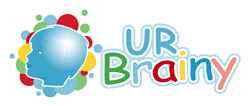If you are looking for bright, colourful worksheets and resources for very young children then urbrainy.com is one site you must visit. They have launched the first of their interactive maths games this week. The site is developing into an excellent resource with plenty of free stuff for young children beginning their understanding of maths. I like the layout, which is bright and modern looking and it seems to be developing by the week.
Subscription for a year is well worthwhile at just £10.00 and I believe that schools can subscribe for a site licence for just £12.00 a year.

## Free Y5 maths worksheet: very large numbers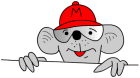Free maths worksheet from mathsblog.co.uk

Once again we have a maths worksheet which needs a very good understanding of place value to answer correctly. Dealing with numbers up to millions is not easy for children: many never succeed in being able to read them correctly.

Whilst the second part of the worksheet requires a calculator, the questions are no less difficult. There are problems with keying in a number such as six million, six hundred thousand or where zeros act as place holders.

When asked to write a number such as six hundred and seventy thousand many children will write something like 600 70 000 ignoring place value (it should read 670 000).

Free Y5 maths worksheet: very large numbers

## mychild

Review of mychild from mathsblog.co.uk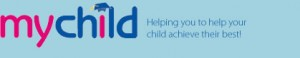Mychild is a website for parents. It offers a huge amount of help including parenting advice, worksheets and resources. Some of these are free but they also offer a subscription service. Whilst this isn’t cheap it does come with some excellent resources across the curriculum and includes magazines and CDs. This costs £86 for one year, but you can get an excellent starter pack of books and CDs for £9.00 (currently valued at over £190) to review for 10 days. If you don’t want it, then by returning within 10 days will ensure that your money is returned. You can’t ask for more than that.

Certainly not everyone’s cup of tea, but many parents do find this a valuable site.

## Free maths worksheet: standard written method of addition of 2-digits

Free maths worksheet from mathsblog.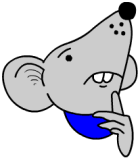This is the first maths worksheet on addition using the standard method.

It must be pointed out that usually 2-digit addition should be tackled ‘in your head’. The example on the first page can be done by adding 50 to 76, making 126 and then subtracting 1 making 125. However, to practise the written method it is often useful to keep to smaller numbers so that children gain confidence with the new method

The method is to add the units first, put the units in the answer, and ‘carry’ the ten into the tens column. Then add the tens. A more detailed description of the method to use is on the first page of the worksheets.

Standard written method of addition of two 2-digit numbers (1)

## Free Y3 maths worksheet: properties of quadrilaterals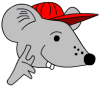Free maths worksheet from mathsblog.co.uk: properties of quadrilaterals.

The term quadrilateral is often  misunderstood and confused with the term rectangle. A quadrilateral is any flat shape which has four straight sides. The angles do not have to be right angles. Rectangles and squares are special cases of quadrilateral.

Look out for quadrilaterals all around you in the real world.

## Free maths worksheet: subtracting 9 from 2-digit numbers

Free maths worksheet from mathsblog.co.uk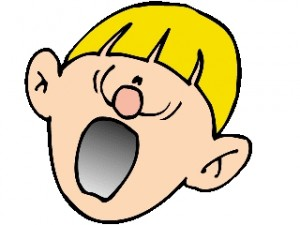Here is another worksheet for subtraction; this time subtracting 9 from 2-digit numbers.

The usual method to do this in your head is to take 10 and then add 1.

So 56 – 9 is done in two steps:

56 – 10 = 46

46 + 1 = 47

56 – 9 = 47

Subtract 9 from a 2-digit number

## Free Y3 maths worksheet: counting on (teens)

Free maths worksheet from mathsblog.co.uk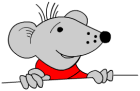Counting on is a very powerful tool when using mental methods of calculating. This worksheet concentrates on counting on a teen number to a number in the hundreds and can be seen as an addition problem (143 + 16).

The usual method of doing this requires the ability to partition (ie know that 16 is 10 + 6) and is in two parts:

1. Count on ten from 143 making 153.

2. Count on 6 from 153 to make 159.

There should not be a need to do this type of question on paper.

Free maths worksheet: counting on (teens)

## Free maths worksheet: adding 7 to a single digit

Free maths worksheet on addition from mathsblog.co.uk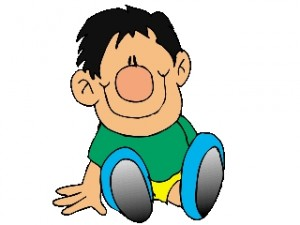Continuing with our series of simple addition of single digits, here are two maths worksheets on adding 7. The ultimate aim is for children to know, off by heart, the answer to any addition of two single digits. This means that they don’t have to think about it, there is no counting on in their heads, they know it and can answer as quickly as if they were asked what their name is.

adding 7 to a single digit (1)

adding 7 to a single digit (2)

## Maths vocabulary for Year 2: multiplication and division

It is in year 2 that multiplication and division really become important. Understanding terms such as ‘lots of’, ‘share equally’ and ‘divide’ are all introduced in a practical sense i.e. children need plenty of opportunities to share items out and to group into sets.
The vocabulary introduced in year 2 concerning multiplication and division of numbers includes:
lots of groups of
times multiply multiplied by multiple of
once twice three times
four times five times up to ten times
times as (long, big etc)
array row column
share equally one each two each three each etc
group in pairs group in tens
equal groups of
divide divided by divided into

Find a larger version of this list in the link below.

Remember that new vocabulary should not be introduced in isolation, but in suitable contexts.

(Taken from Mathematical Vocabulary Book DfEE)
Maths vocabulary: multiplication and division

## Reception maths worksheet: counting and matching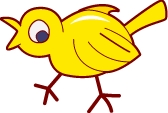Here is the second in a series of maths worksheets for very young children dealing with counting and matching. It involves drawing lines joining sets with the same number of birds and worms and comes from the fabulous collection of resources from urbrainy.com.

Counting and matching birds p1